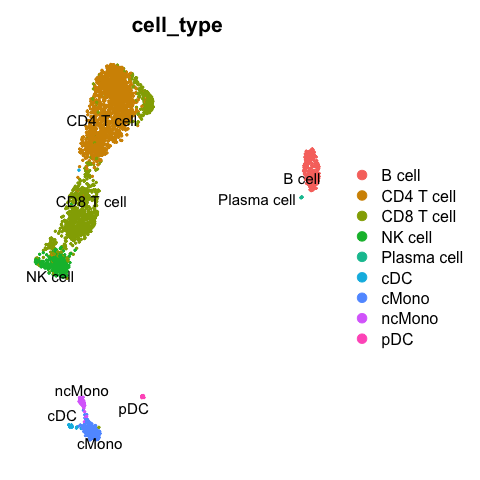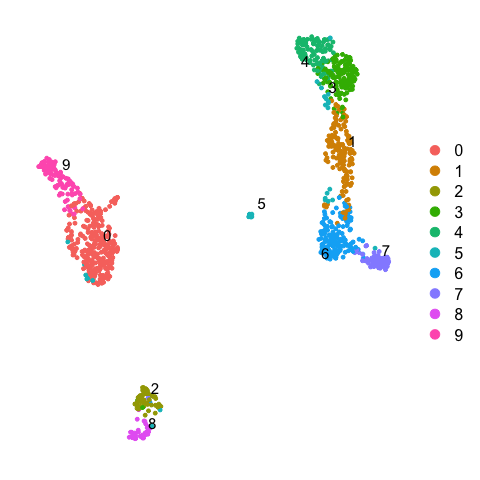## Celltype prediction

Celltype prediction can either be performed on indiviudal cells where each cell gets a predicted celltype label, or on the level of clusters. All methods are based on similarity to other datasets, single cell or sorted bulk RNAseq, or uses known marker genes for each celltype.

We will select one sample from the Covid data, `ctrl_13` and predict celltype by cell on that sample.

Some methods will predict a celltype to each cell based on what it is most similar to even if the celltype of that cell is not included in the reference. Other methods include an uncertainty so that cells with low similarity scores will be unclassified. There are multiple different methods to predict celltypes, here we will just cover a few of those.

Here we will use a reference PBMC dataset from the `scPred` package which is already a Seurat object with counts. And we will test classification based on label transfer using the function `TransferData` in the Seurat package and the `scPred` method. Finally we will use gene set enrichment predict celltype based on the DEGs of each cluster.

## Covid-19 data

First, lets load required libraries and the saved object from the clustering step. Subset for one patient.

``````suppressPackageStartupMessages({
library(Seurat)
library(dplyr)
library(cowplot)
library(ggplot2)
library(pheatmap)
library(rafalib)
library(scPred)
})``````
``````# load the data and select 'ctrl_13` sample
ctrl = alldata[, alldata\$orig.ident == "ctrl_13"]

# set active assay to RNA and remove the CCA assay
ctrl@active.assay = "RNA"
ctrl[["CCA"]] = NULL
ctrl``````
``````## An object of class Seurat
## 18121 features across 1139 samples within 1 assay
## Active assay: RNA (18121 features, 0 variable features)
##  6 dimensional reductions calculated: umap, tsne, harmony, umap_harmony, scanorama, umap_scanorama``````

## Reference data

Then, load the reference dataset with annotated labels. Also, run all steps of the normal analysis pipeline with normalizaiton, variable gene selection, scaling and dimensionality reduction.

``````reference <- scPred::pbmc_1

reference``````
``````## An object of class Seurat
## 32838 features across 3500 samples within 1 assay
## Active assay: RNA (32838 features, 0 variable features)``````

## Rerun analysis pipeline

Here, we will run all the steps that we did in previous labs in one go using the `magittr` package with the pipe-operator `%>%`.

``````reference <- reference %>%
NormalizeData() %>%
FindVariableFeatures() %>%
ScaleData() %>%
RunPCA(verbose = F) %>%
RunUMAP(dims = 1:30)``````
``DimPlot(reference, group.by = "cell_type", label = TRUE, repel = TRUE) + NoAxes()``Run all steps of the analysis for the ctrl sample as well. Use the clustering from the integration lab with resolution 0.5.

``````# Set the identity as louvain with resolution 0.3
ctrl <- SetIdent(ctrl, value = "CCA_snn_res.0.5")

ctrl <- ctrl %>%
NormalizeData() %>%
FindVariableFeatures() %>%
ScaleData() %>%
RunPCA(verbose = F) %>%
RunUMAP(dims = 1:30)``````
``DimPlot(ctrl, label = TRUE, repel = TRUE) + NoAxes()``# Seurat label transfer

First we will run label transfer using a similar method as in the integration exercise. But, instad of CCA the default for the ’FindTransferAnchors` function is to use “pcaproject”, e.g. the query dataset is projected onto the PCA of the reference dataset. Then, the labels of the reference data are predicted.

``````transfer.anchors <- FindTransferAnchors(reference = reference, query = ctrl, dims = 1:30)
predictions <- TransferData(anchorset = transfer.anchors, refdata = reference\$cell_type,
dims = 1:30)
``DimPlot(ctrl, group.by = "predicted.id", label = T, repel = T) + NoAxes()``